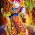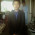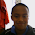## Wednesday, 18 March 2015

### This is a problem the Triangles group could not solve on Tuesday and the students who did not go to cricket are so close to solving.

I have 3 dogs, if I add their ages together I get 15. If I multiply their ages I get 45. How old are my dogs?

1.I have solved it. When I tried hard to work it out I could't get it when I just let it play in my mind I solved it. Good luck all.

2.This comment has been removed by the author.

3.Taki the first part is right but have a look at the multiplication. You are on the right track though.

4.1 + 5 + 9 = 15
1 x 5 x 9 = 45

1.How do you understand so much?

5.Is the anwser 5x9=45

1.Yes but there are 3 dogs and each dog has an age. When I was working the problem out I started from 45. So I went ? X ? = 45? and I knew it is 9X5 = 45 and I thought how about the other dog then I put 1 because 45X1 is still 45. So the ages of the dogs are 1, 5, and 9. Do you agree Samuel?

2.Now I agree but I said 9x5

6.Yes Taki that is correct. Awesome.

7.I still don't agree I wanna solve it another way

1.Ok Samuel lets see if there is another solution to the problem. Good luck.

2.Samuel keep thinking.

8.Miss is the answer 45?
1+5+9= 15
9x5=45

9.1+5+9=15
1x5x9=45

1.Yes that is correct.

10.Correct Aarmione :)

11.How did you get it taki I can't understand

1.By thinking